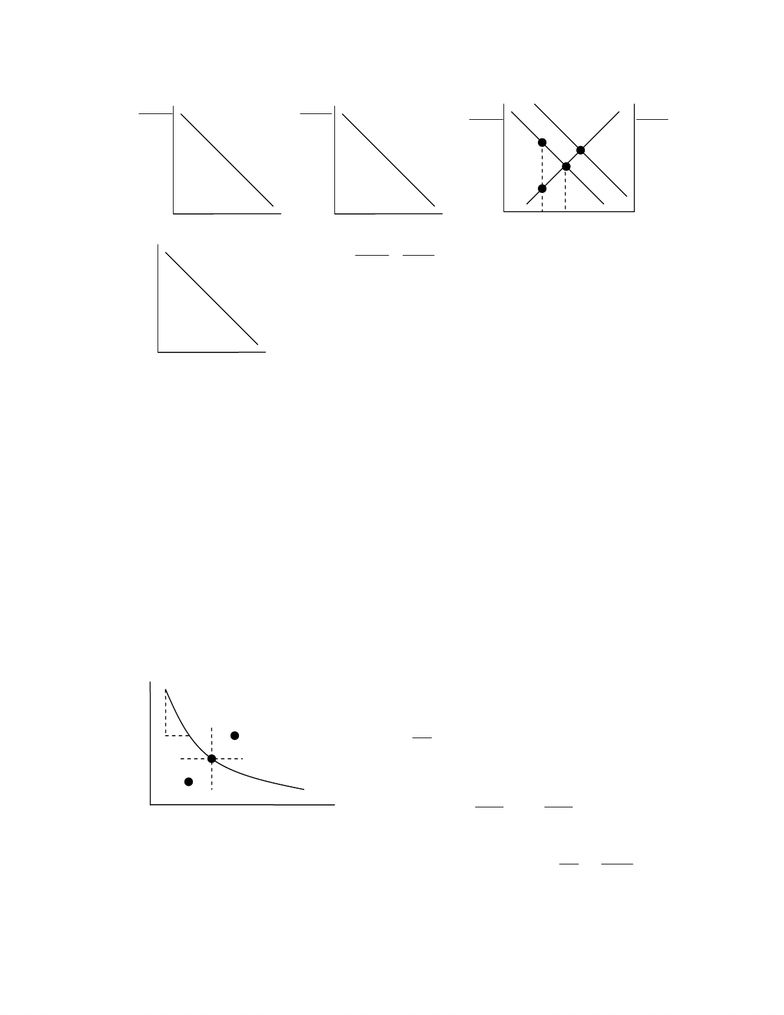# Summary notes 1

194 views21 pages
School
Department
Course
ProfessorECO200Y1a - Consumer Theory.doc
Page 1 of 21
Utility Theory
ONE GOOD UTILITY THEORY
Cardinal measure of utility precise, accurate, numerical measurement.
Assume one good X:
Total Utility =
(
)
XTU.
Marginal Utility =
( )
(
)
X
X
X
=TU
MU.
Diminishing marginal utility Can get too much of a good thing..
Example: Deriving Demand
MU drives D diminishing marginal utility leads to a
negatively sloped D.
Constant marginal utility of money constant value
everywhere.
CONSUMER SURPLUS
The difference of the value of the commodity and how much the consumer spend on it.
Consumer surplus comes about because every unit is the same price, but consumers value each unit
differently.
Example
Given qp 212
=
, at 2
=
p,
( )( )
252125
2
1==CS.
Note the \$5 difference is due to the extra area from the triangles.
TWO GOOD UTILITY FUNCTION
(
)
(
)
(
)
YXYXTUTU,TU
+
=
X and Y are not related goods.
Equilibrium:
Y
Y
X
X
P
MU
P
MU=
6 5 4
6 5 4
2
4
4
6
8
10
2
D1
MU
Q
Q
MU
P
www.notesolution.com
Unlock document

This preview shows pages 1-3 of the document.
Unlock all 21 pages and 3 million more documents.ECO200Y1a - Consumer Theory.doc
Page 2 of 21
Y
Y
X
X
P
MU
P
MU=.
If PX, then QX.
Indifference Theory
INDIFFERENCE CURVES
Definition
All points with the same level of satisfaction.
Assumptions
Consumers are able to rank given A and B, they are able to pick the preferred one.
Transitivity/rational/consistent if A > B > C, then A > C.
More is preferred to less 0
>
MU.
Derivation
B is preferred to A (more of both goods) and A is
preferred to C (more of both goods), so B and C are
not on IC IC negatively sloped.
<
FC
C,0.
Typically non-linear:
C
F
FC
MU
MU
F
C
MUFMUC
F
TU
F
C
TU
C
TUTU
=
=+
=
+
=
+
0
0
0F todue C todue
.
A
B
C
F
C
IC
C
F
X
X
P
MU
X
Y
Y
P
MU
Y
xxxx
xxxx
xxxx
xxxx
xxxx
xxxx
xxxx
xxxx
A
B
MUX/PX
MUY/PY
X
X
P
MU
Y
Y
P
MU
X
Y
PX
Q
D
www.notesolution.com
Unlock document

This preview shows pages 1-3 of the document.
Unlock all 21 pages and 3 million more documents.ECO200Y1a - Consumer Theory.doc
Page 3 of 21
MRS: Willingness to trade on IC (holding TU constant)
C
F
CFMU
MU
MRS=
/.
IC convex because of marginal utility diminishing marginal rate of substation.
ICs cant cross there is one and only one IC through any point.
Special Cases
F and C are perfect substitutes.
MRS constant.
IC is linear slope of IC = MRS.
F is a neutral good (adds no satisfaction).
IC is horizontal.
C is a neutral good (adds no satisfaction).
IC is vertical.
F and C must be consumed in fixed proportions.
Increases in only one good adds no satisfaction.
<
C
MU).
Additional units of C will decrease satisfaction.
12 ICIC
>
.
F
C
IC2
IC1
F
C
IC2
IC1
F
C
IC2
IC1
F
C
IC2
IC1
F
C
IC2
IC1
www.notesolution.com
Unlock document

This preview shows pages 1-3 of the document.
Unlock all 21 pages and 3 million more documents.